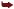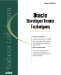# Operations on a Tree Item

This section highlights the techniques for performing various operations on tree items. It begins by cautioning the developer with the effect of specifying an ORDER BY clause for a hierarchical tree query and then describing the technique for dynamically populating a tree item using a record group or a query. Node operations such as single click, double-click, expand, expand all, collapse, collapse all, and search on a particular node are described. Finally, techniques for adding and deleting sub-trees and singular nodes are presented.

Caution Regarding Ordering Tree Item Data

Be careful in specifying an ORDER BY clause for a tree item query. The ORDER BY takes precedence over the hierarchy. So the child nodes of a parent node might appear above the parent node if the ORDER BY order precedes the TREE HIERARCHY order.

Dynamically Populating a Tree

A tree can be dynamically populated by using record groups and using query text. Two ways to dynamically populate a tree are as follows :

• Specify an initial data query in the tree item properties. Use FTREE.POPULATE_TREE to populate the tree with data contained in the data query. This is illustrated in the previous section "Creating a Tree Item."
• Populate the tree item using a record group created programmatically. Use FTREE.SET_TREE_PROPERTY to do this. A call to this built-in replaces the existing tree data with the data contained in the record group and causes the tree to display the new data. The following code illustrates this concept:

```DECLARE

rg_id RECORDGROUP;

ret_code NUMBER;

BEGIN

/* If the record group already exists, delete it. */

g_id := FIND_GROUP(''RG_TREE'');

IF NOT ID_NULL(rg_id) THEN

DELETE_GROUP(rg_id);

END IF;

/* Create the record group using a query */

rg_id := CREATE_GROUP_FROM_QUERY(''RG_TREE'',

''SELECT -1, LEVEL, ename, NULL, TO_CHAR(empno)

FROM emp

/* Populate the record group using data from the query with which it was

/created */

ret_code := POPULATE_GROUP(rg_id);

/* If the above populate is a success then set tree property as follows */

IF (ret_code = 0) THEN

FTREE.SET_TREE_PROPERTY(''htree.htree'',FTREE.RECORD_GROUP, rg_id);

;

END;
```

The following points are to be noted here:

• The record group has to be created programmatically and has to be a query record group with a SELECT statement having a START WITH and CONNECT BY clause.
• The query for the record group has to conform to the five column query structure of the tree initial data query.
• There is no need to use FTREE.POPULATE_TREE. Using FTREE.SET_TREE_PROPERTY automatically populates the tree with the new data and displays it.
• The data source of a tree query can be of type record group or query text. It is specified by either the constant FTREE.RECORD_GROUP or FTREE.QUERY_TEXT.

Tip

The current data source of a tree can be obtained as follows:

```FTREE.GET_TREE_PROPERTY(item_id, DATASOURCE);
```

Selection of a Node

Selecting a node is the most important step in using a tree item. Selection of a node refers to single-clicking a particular node of the tree. Generally an action pertaining to the node value selected is initiated when a node is selected by means of a single-click .

In our example tree, when the user selects (single-clicks) an ENAME in the tree, the other related details pertaining to the employee name are displayed. To do this, write a WHEN-TREE-NODE-SELECTED trigger with calls to :SYSTEM.TRIGGER_NODE and GET_TREE_NODE_PROPERTY. The system variable :SYSTEM.TRIGGER_NODE points to the node the user single-clicks. A call to the built-in FTREE.GET_TREE_NODE_PROPERTY (as shown in the code below) gives the value contained in the node selected. The code is as follows:

```
DECLARE

item_id ITEM;

node_ret_val VARCHAR2(32767);

BEGIN

item_id := FIND_ITEM(''TREE_BLOCK.HTREE'');

IF NOT ID_NULL(item_id) THEN

/* Get the node value corresponding to the node selected. The specific node selected isobtained by the system variable :SYSTEM.TRIGGER_NODE */

node_ret_val := FTREE.GET_TREE_NODE_PROPERTY(item_id, :SYSTEM.TRIGGER_NODE,FTREE.NODE_VALUE);

/* Get the employee details corresponding to the node value so obtained above. This isdone by setting the default where clause of the EMP block followed by an EXECUTE_QUERY inthe block */

SET_BLOCK_PROPERTY(''EMP'', DEFAULT_WHERE,

''WHERE empno = ''TO_NUMBER(node_ret_val));

GO_BLOCK(''EMP'');

EXECUTE_QUERY(ALL_RECORDS);

END IF;

END;
```

Double-clicking a Node

Double-clicking a tree node activates a user-specified action. This is in conformity with the standard Windows functionality: single-click for selection and double-click for action. An example of this is Expand All or Collapse All for the currently selected node.

Write a WHEN-TREE-NODE-ACTIVATED trigger to perform the desired action. The use of this trigger is illustrated in the following sections Expanding All Nodes and "Collapsing All Nodes."

Expanding a Node

This is done to expand the current node to display depth one level below.

Note

There is a difference between selection and expansion of a node. Selection is highlighting the node by clicking on the node name, whereas expansion is selection done by clicking on the icon next to the name. In other words, it's the difference between clicking on a and the + icon to the left of it in the object navigator.

Collapsing a Node

This is done to collapse the current node to a height one level above. The functions EXPAND and COLLAPSE are controlled by default by the WHEN-TREE-NODE-EXPANDED trigger.

The following code toggles between expanding and collapsing depending on the current state of the node:

```
DECLARE

item_id ITEM;

node_ret_val VARCHAR2(32767);

this_node FTREE.NODE;

BEGIN

/* Get the internal id of the tree item */

item_id := FIND_ITEM(''TREE_BLOCK.HTREE'');

IF NOT ID_NULL(item_id) THEN

/* Get the currently selected node */

this_node := GET_TREE_SELECTION(item_id, 1);

IF NOT FTREE.ID_NULL(this_node) THEN

/* If internal id is not null, switch this node''s state to the opposite of its currentstate */

IF (FTREE.GET_TREE_NODE_PROPERTY(item_id, this_node, FTREE.NODE_STATE) =FTREE.EXPANDED_NODE THEN

FTREE.SET_TREE_NODE_PROPERTY(item_id, this_node, FTREE.NODE_STATE,FTREE.COLLAPSED_NODE);

ELSIF (FTREE.GET_TREE_NODE_PROPERTY(item_id, this_node, FTREE.NODE_STATE) =FTREE.COLLAPSED_NODE THEN

FTREE.SET_TREE_NODE_PROPERTY(item_id, this_node, FTREE.NODE_STATE,FTREE.EXPANDED_NODE);

END IF;

END IF;

END IF;

END;
```

The user has to select by clicking on the node (thus highlighting it). The code gets the first such selected node and toggles between expanded and collapsed states. Note that a WHEN-BUTTON-PRESSED trigger can also be used to execute this code.

Expanding All Nodes

This is done to expand the current node to display all levels below to the atomic level.

We will use the following procedure to do the job:

```
PROCEDURE p_expand_or_collapse_all

(htree VARCHAR2, exp_or_coll VARCHAR2, ret_cd OUT NUMBER)

IS

item_id Item;

current_node FTREE.Node;

starting_node_level NUMBER;

current_node_level NUMBER;

BEGIN

item_id := FIND_ITEM(htree);

IF NOT ID_NULL(item_id) THEN

/* Get the current node and its depth */

current_node := :SYSTEM.TRIGGER_NODE;

starting_node_level := FTREE.GET_TREE_NODE_PROPERTY(item_id, current_node,FTREE.NODE_DEPTH);

/* Traverse down thr tree till the atomic node is reached. This atomic node is

/relative to the current node. While traversing expand each collapsed node in

/the path. We are sure that we have reached the atomic if there are no more

/nodes or we have reached a node whole depth is the same as the starting node

/ level */

LOOP

IF FTREE.ID_NULL(current_node) OR (current_node_level = starting_node_level) THEN

EXIT;

ELSE

/* The literal ''X''specifies that the operation is that od expansion. The

/literal ''C''signifies collapsing */

IF exp_or_coll = 'X'THEN

IF FTREE.GET_TREE_NODE_PROPERTY(item_id, current_node, FTREE.NODE_STATE) =FTREE.COLLAPSED_NODE THEN

FTREE.SET_TREE_NODE_PROPERTY(item_id, current_node, FTREE.NODE_STATE,FTREE.EXPANDED_NODE);

END IF;

ELSIF exp_or_coll = 'C'THEN

IF FTREE.GET_TREE_NODE_PROPERTY(item_id, current_node, FTREE.NODE_STATE) =FTREE.EXPANDED_NODE THEN

FTREE.SET_TREE_NODE_PROPERTY(item_id, current_node, FTREE.NODE_STATE,FTREE.COLLAPSED_NODE);

END IF;

END IF;

/* Reset the current node and the current node level. The immediately traversed

/ node becomes the current node and its depth becomes the current node level */

current_node := FTREE.FIND_TREE_NODE (htree, '', search_type=>FTREE.FIND_NEXT,search_point =>current_node);

current_node_level := FTREE.GET_TREE_NODE_PROPERTY (item_id, current_node,FTREE.NODE_DEPTH);

END IF;

END LOOP;

END IF;

END;
```

Write a WHEN-TREE-NODE-ACTIVATED trigger to expand all nodes of the current node. This expansion to the current node as the root node is as follows:

```WHEN-TREE-NODE-ACTIVATED

DECLARE

retcd NUMBER;

BEGIN

p_expand_or_collapse_all('TREE_BLOCK.HTREE','X', retcd);

IF (retcd <> 0) THEN

MESSAGE('ERR: Expand All/Collapse All failed');

RAISE FORM_TRIGGER_FAILURE;

END IF;

END;
```

Collapsing All Nodes

This is done to collapse the current node to a height of all levels above ”that is, to the current node level.

The procedure described in the previous section can be used to collapse all nodes with the current node as the root node:

```WHEN-TREE-NODE-ACTIVATED

DECLARE

retcd NUMBER;

BEGIN

p_expand_or_collapse_all('TREE_BLOCK.HTREE','C', retcd);

IF (retcd <> 0) THEN

MESSAGE('ERR: Expand All/Collapse All failed');

RAISE FORM_TRIGGER_FAILURE;

END IF;

END;
```

Note that the literal C is passed to indicate the operation of collapsing.

Tip

There is no automatic Expand All or Collapse All available at runtime for a tree item. The default toggle operation of expand/collapse can be used to toggle between these two node states.

Finding a Particular Node

Searching for a particular node is possible based on either the node value or the node label. This might be required when one is simulating drill-down LOVs using tree items. However, this search is not based on wildcard characters , unlike the one in a Forms-supplied LOV. You use FTREE.FIND_TREE_NODE with the search_string, search_type, search_by, search_root, and start_point specified:

```
FTREE.FIND_TREE_NODE(item_id, search_string, search_type, search_by, search_root,start_point);
```

The search_string is the value of the node or the label of the node depending on whether the search_by (the third parameter after the item_id) is node value or node label ( FTREE.NODE_VALUE or FTREE.NODE_LABEL ).

The search_type is the type of search: whether to find the next successive node (irrespective of child or sibling) or the next child node. Valid values are FTREE.FIND_NEXT for the former and FTREE.FIND_NEXT_CHILD for the latter.

Consider the tree shown in Figure 9.2.

Let the search_root be the node identified by ROOT NODE of the tree specified as FTREE.ROOT_NODE.

The following code

```
DECLARE

item_id ITEM;

current_node FTREE.NODE;

starting_node_level NUMBER;

searched_node_label VARCHAR2(15);

BEGIN

item_id := FIND_ITEM('TREE_BLOCK.HTREE');

IF ID_NULl(item_id) THEN

MESSAGE('Invalid Tree Item');

RAISE FORM_TRIGGER_FAILURE;

END IF;

current_node := FTREE.FIND_TREE_NODE(item_id,'', FTREE.FIND_NEXT, FTREE.NODE_VALUE,FTREE.ROOT_NODE, FTREE.ROOT_NODE);

searched_node_label := FTREE.GET_TREE_NODE_PROPERETY(item_id, current_node,FTREE.NODE_LABEL);

END;
```

yields KING as the next node of the tree ROOT NODE.

Tip

Specify a NULL search string to locate the successive or next successive child node without basing the search on a value.

Replacing FTREE.FIND_NEXT by FTREE.FIND_NEXT_CHILD also yields ADAMS. This is because, for the tree ROOT NODE, both the next node and the next child node are ADAMS, starting at the root.

Next we find the node identified by the label ALLEN. To do this, we replace the NULL value for the search string by the string ALLEN:

```
DECLARE

item_id ITEM;

current_node FTREE.NODE;

starting_node_level NUMBER;

searched_node_label VARCHAR2(15);

BEGIN

item_id := FIND_ITEM('TREE_BLOCK.HTREE');

IF ID_NULl(item_id) THEN

MESSAGE('Invalid Tree Item');

RAISE FORM_TRIGGER_FAILURE;

END IF;

current_node := FTREE.FIND_TREE_NODE(item_id, 'ALLEN', FTREE.FIND_NEXT,FTREE.NODE_VALUE, FTREE.ROOT_NODE, FTREE.ROOT_NODE);

searched_node_label := FTREE.GET_TREE_NODE_PROPERTY(item_id, current_node,FTREE.NODE_LABEL);

IF (searched_node_label IS NOT NULL) THEN

MESSAGE(searched_node_label, ACKNOWLEDGE);

END IF;

END;
```

The nodes label obtained is displayed to verify the fact. The search_root and the search_point are both FTREE.ROOT NODE in this case.

Next we perform the above search with ALLEN as the root node. As evident from the tree diagram in Figure 9.2, ALLEN has no child node. The above code is modified as follows:

```
DECLARE

item_id ITEM;

current_node FTREE.NODE;

starting_node_level NUMBER;

searched_node_label VARCHAR2(15);

BEGIN

item_id := FIND_ITEM('TREE_BLOCK.HTREE');

IF ID_NULl(item_id) THEN

MESSAGE('Invalid Tree Item');

RAISE FORM_TRIGGER_FAILURE;

END IF;

current_node := FTREE.FIND_TREE_NODE(item_id, 'ALLEN', FTREE.FIND_NEXT,FTREE.NODE_LABEL, FTREE.ROOT_NODE, FTREE.ROOT_NODE);

current_node := FTREE.FIND_TREE_NODE(item_id,'', FTREE.FIND_NEXT, FTREE,NODE_LABEL,current_node, current_node);

searched_node_label := FTREE.GET_TREE_NODE_PROPERTY(item_id, current_node,FTREE.NODE_LABEL);

IF (searched_node_label IS NOT NULL) THEN

MESSAGE(searched_node_label, ACKNOWLEDGE);

END IF;

END;
```

We get WARD as the resulting node label.

Next we replace FTREE.FIND_NEXT with FTREE.FIND_NEXT_CHILD in the call to FTREE.FIND_TREE_NODE. We get a null value.

Tip

The search type is always from the starting point and relative to the search root.Oracle Developer Forms Techniques
ISBN: 0672318466
EAN: 2147483647
Year: 2005
Pages: 115